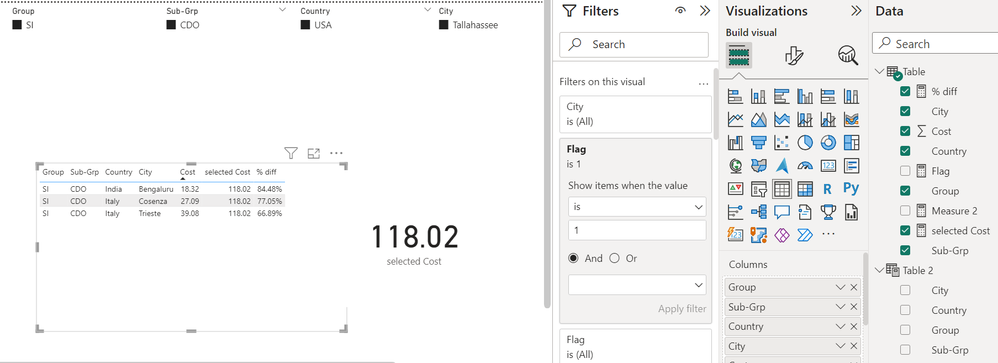cancel
Showing results for
Did you mean:

Fabric is Generally Available. Browse Fabric Presentations. Work towards your Fabric certification with the Cloud Skills Challenge.Regular Visitor

## Store slicer selections from a user and compare the selection against others

 Group Sub-Grp Country City Cost SI CDO USA Tallahassee 118.02 SI CDO Germany Berlin 73.22 SI CDO Italy Trieste 39.08 SI CDO Italy Cosenza 27.09 SI CDO France Strasbourg 67.33 SI CDO India Bengaluru 18.32

In power BI, there will be 4 slicers, Group, Track, Country and City. If an user selects SI, CDO, USA and Tallahassee, cost 118.02 will be shown on a card. Now, I need to show in another table visual what are the best 3 lowest cost city compared to the user selection and show the % difference. Output should look like:

 Group Sub-Grp Country City Actual Cost User selected Cost % diff SI CDO India Bengaluru 18.32 118.02 84.48% SI CDO Italy Cosenza 27.09 118.02 77.05% SI CDO Italy Trieste 39.08 118.02 66.89%

Please suggest how to achieve this.

1 ACCEPTED SOLUTIONCommunity Support

Hi  @Bari ,

Here are the steps you can follow：

1. Create calculated table.

Create a slicer table that has no relationship to the main table.

``````Table 2 =
SUMMARIZE(
'Table','Table'[Group],'Table'[Sub-Grp],'Table'[Country],'Table'[City])``````2. Create measure.

``````selected Cost =
var _selectgroup=SELECTEDVALUE('Table 2'[Group])
var _selectsubgroup=SELECTEDVALUE('Table 2'[Sub-Grp])
var _selectcountry=SELECTEDVALUE('Table 2'[Country])
var _selectcity=SELECTEDVALUE('Table 2'[City])
return
SUMX(
FILTER(ALL('Table'),
'Table'[Group]=_selectgroup&&'Table'[Sub-Grp]=_selectsubgroup&&'Table'[Country]=_selectcountry&&'Table'[City]=_selectcity),[Cost])``````
``````Flag =
var _selectgroup=SELECTEDVALUE('Table 2'[Group])
var _selectsubgroup=SELECTEDVALUE('Table 2'[Sub-Grp])
var _rank=
RANKX(
FILTER(ALL('Table'),
'Table'[Group]=_selectgroup&&'Table'[Sub-Grp]=_selectsubgroup),CALCULATE(SUM('Table'[Cost])),,ASC)
return
IF(
_rank<=3,1,0)``````
``````% diff =
DIVIDE(
[selected Cost]-SUM('Table'[Cost]),[selected Cost])``````

3. Place [Flag]in Filters, set is=1, apply filter.4. Result:Best Regards,

Liu Yang

If this post helps, then please consider Accept it as the solution to help the other members find it more quickly

7 REPLIES 7Community Support

Hi  @Bari ,

Here are the steps you can follow：

1. Create calculated table.

Create a slicer table that has no relationship to the main table.

``````Table 2 =
SUMMARIZE(
'Table','Table'[Group],'Table'[Sub-Grp],'Table'[Country],'Table'[City])``````2. Create measure.

``````selected Cost =
var _selectgroup=SELECTEDVALUE('Table 2'[Group])
var _selectsubgroup=SELECTEDVALUE('Table 2'[Sub-Grp])
var _selectcountry=SELECTEDVALUE('Table 2'[Country])
var _selectcity=SELECTEDVALUE('Table 2'[City])
return
SUMX(
FILTER(ALL('Table'),
'Table'[Group]=_selectgroup&&'Table'[Sub-Grp]=_selectsubgroup&&'Table'[Country]=_selectcountry&&'Table'[City]=_selectcity),[Cost])``````
``````Flag =
var _selectgroup=SELECTEDVALUE('Table 2'[Group])
var _selectsubgroup=SELECTEDVALUE('Table 2'[Sub-Grp])
var _rank=
RANKX(
FILTER(ALL('Table'),
'Table'[Group]=_selectgroup&&'Table'[Sub-Grp]=_selectsubgroup),CALCULATE(SUM('Table'[Cost])),,ASC)
return
IF(
_rank<=3,1,0)``````
``````% diff =
DIVIDE(
[selected Cost]-SUM('Table'[Cost]),[selected Cost])``````

3. Place [Flag]in Filters, set is=1, apply filter.4. Result:Best Regards,

Liu Yang

If this post helps, then please consider Accept it as the solution to help the other members find it more quicklyRegular Visitor

@v-yangliu-msft one more question for you. I have different groups and sub-groups for the same countries and cities in my data. How to keep the slicer selection for group and sub-group constant and show the ranking of city based on the slicer selection of group and sub-group?Regular Visitor

@v-yangliu-msft Thank you so much for your help. It worked.Regular Visitor

Hi @JoeBarry , both the costs are there in the same table. If an user selects one city, we should be able to calculate the difference in cost with the other cities in that table.

``````VAR _SelCost = SUM(Table1[Cost])
VAR _Cost = SUM(Table1[Cost)``````Solution Sage

Hi @Bari

For the 2nd visual, turn off the interaction between the Country and City and the 2nd table

Highlight the Country Slicer, in the ribbion a Format tab will appear. Click on Edit interactions. While the slicer is highlighted, turn off the interaction in the 2nd table by clicking on this iconrepeat for the city slicer.

Within the table open the filter pane right. Within the cost part go to filter type and choose Top N and add the Cost measure to the By value field. Then in Show items choose bottom and enter 3 in the empty field and then apply

This should do it for you.

Thanks

Joe

If this post helps, then please Accept it as the solutionRegular Visitor

Hi @JoeBarry , thank you for your reply. I alredy turned off the interaction. The issue is I could not store the user selected value as a reference point in my table which is 118.02 in the example. and then I need to show the dirrence between 118.02 and 18.32 which is 84.48%. When I a trying to place the user_selected_value measure in the table, instead of 118.02 it is giving me 18.32 only for Bangalore.Solution Sage

This will work if one City is filtered.

Create a measure

``````Cost Difference % =
VAR _SelCost = SUM(Table1[Cost])
VAR _Cost = SUM(Table2[Cost)
VAR _Diff = _SelCost - _Cost

RETURN
DIVIDE(_Diff , _SelCost)``````

Convert to % and add to VisualAnnouncements#### Power BI Monthly Update - November 2023

Check out the November 2023 Power BI update to learn about new features.#### Fabric Community News unified experience

Read the latest Fabric Community announcements, including updates on Power BI, Synapse, Data Factory and Data Activator.#### The largest Power BI and Fabric virtual conference

130+ sessions, 130+ speakers, Product managers, MVPs, and experts. All about Power BI and Fabric. Attend online or watch the recordings.Top Solution Authors
Top Kudoed Authors
Users online (4,500)